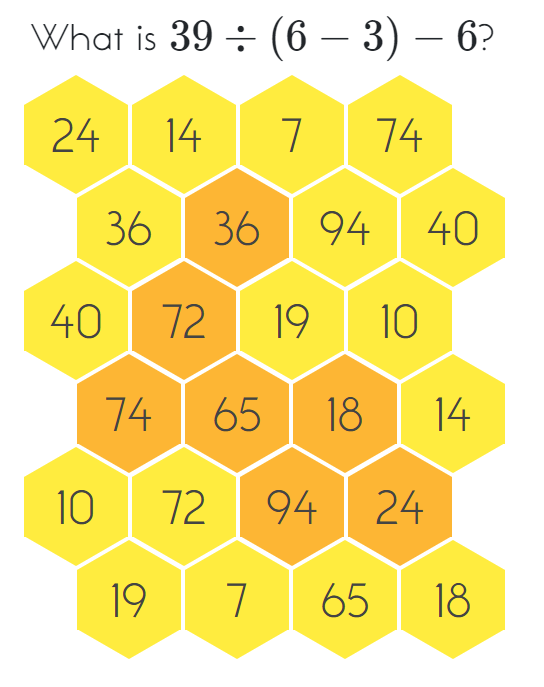MM Practice
×
Multiplication
Division
Place Value
Fractions & Decimals
Measurement
Statistics & Probability
Pre-Algebra
Money
Kindergarten
Geometry

# Hexingo - Order of Operations GameOnline practice for grades 3-8

Practice the order of operations in this fun game! Get four in a row to win.

You can choose from five different operations to practice: addition, subtraction, multiplication, division, and exponents. The game even allows you to choose only addition, only sutraction, or only multiplication, to practice adding/subtracting/multiplying many numbers.

Include the following operations:
Subtraction
Multiplication
Division
Exponents
Parentheses

Number of operations:

Screenshot: